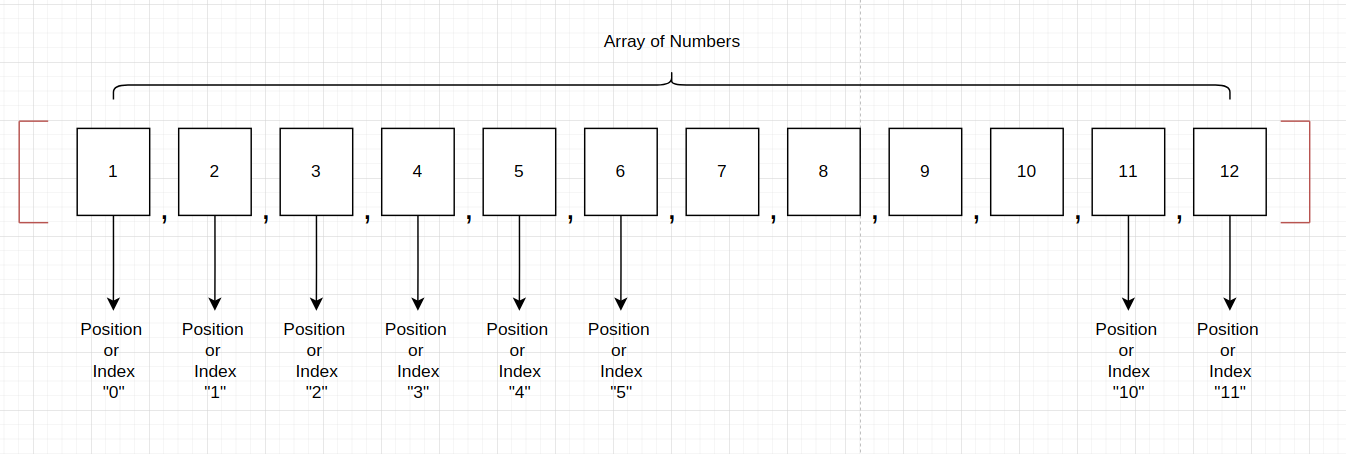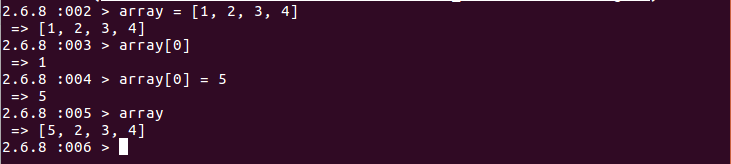Back-end Engineering Articles

I write and talk about backend stuff like Ruby, Ruby On Rails, Databases, Testing, Architecture / Infrastructure / System Design, Cloud, DevOps, Backgroud Jobs, and more...

@daniel_moralesp

2019-06-21

## Introduction to Ruby Data Structures and Complexity Analysis

Now we're going to enter a field of computer science that will help you understand even better the things we've been learning in the past blog posts: Data Structures and Complexity Analysis.

But why is this so important right now? It is fair to say that we are not going so deeper in these concepts (at least not for now), but these will become the fundamental basis of our skills as software developers in Ruby. We're going to understand the importance of this basic knowledge.

The other reason it is so essential to understand data structures and complexity analysis right now is that we'll need to start solving problems, challenges, or algorithms in Ruby to practice what we've learned so far. Of course, we already know Ruby's basic data types and data structures, just like Arrays, Strings, or Hashes. And we know how to encapsulate code inside functions or go over a control flow with IF statements. The missed step is the practice: solving coding problems. But before that, we've to understand how to measure the best solution for a given problem. So let's begin.

# Data Structures

We've already seen 3 data structures with Ruby:

But what is a data structure? In computer science, a data structure is a data organization, management, and storage format that enables efficient access and modification. More precisely, a data structure is a collection of data values, their relationships, and the functions or operations that can be applied to the data. For a deeper theoretical definition, please hit this link.

This definition leads us to understand more in detail about Arrays and Hashes. Remember that both of them are containers of information. For instance, do you remember our image about Arrays? If not too well, here it is again.Here we have a container of Integers in ruby. This Array helps us organize different integers, assigning them order and an index, allowing us to manage and store these integers. Also, it enables us to access and modify its elements. So we have, for instance, simple operations:

```2.6.8 :002 > array = [1, 2, 3, 4]
=> [1, 2, 3, 4]
2.6.8 :003 > array
=> 1
2.6.8 :004 > array = 5
=> 5
2.6.8 :005 > array
=> [5, 2, 3, 4]```Explanation:
• * In the first line, we've created the data structure (Array)
• * Then we access the first element of the data structure with Array 
• * After that, we modify the first element of the data structure array = 5
• * Finally, we printed out the final data structure. All of this means that we organize the data structure at our will.

The same thing happens with Hashes. We can create, access, modify and organize our Hashes at our will. You just need to follow the syntax given by Ruby.

# But why is this important for us as Ruby developers?

As software developers in Ruby, we have to become problem solvers; this means that we've to leave aside (at least for a while) our theoretical knowledge and apply all of these concepts to solve real-world problems. To do that, we've to know about how to code, but at the same time, we've to know about the data structures themselves because it will help us to do things better.

However, data structures are an entire field of study by itself. What we need at this moment is to know how to choose the best data structure to solve a given problem. Typically, just a couple of data structures (20%) generally help us solve 80% of the problems. Choose the best data structure becomes with another vital concept: Complexity Analysis.

# Complexity Analysis

It's essential for us as developers to understand that there are different ways to solve the same problem. Probably you found yourself (or you'll find yourself) in that situation where you are thinking in various ways to solve the same problem (or you think by yourself that it could be a better solution). Sure enough, there will be other solutions. The questions are:

• * It's worth pursuing that solution?
• * Which solution is better?
• * How can I measure and choose the best solution?

Here is where Complexity Analysis comes to help us. In computer science, the Complexity Analysis of an algorithm is the number of resources required to run it. Particular focus is given to time and memory requirements. The complexity of a problem is the complexity of the best algorithms that allow solving the problem.

Complexity doesn't mean that the more complex algorithm will win. Actually, the simpler one wins (if the algorithm takes less time and less space, that means simpler). So complexity is just the name for the analysis. But what we really need to measure here is the Time and Space consumed by the solution.

Time Complexity is usually related to the number of seconds or minutes that the solution takes to run successfully. Space Complexity is related to the amount of memory needed to run the algorithm. So the key questions are: How fast is the solution? How much memory does it consume? Of course, the best solution is the one that has low numbers in both questions.

However, this also depends on the use case itself. Sometimes we would prefer to execute in less time. It doesn't matter the memory consumption. Or on the other hand, you probably give more importance to the memory resources than the time it takes to execute. Or you can tolerate a bit of delay in both if they are running in a tolerable time and space complexity.

All of this means that choosing the best algorithm to solve a problem is in part about the use case, the priorities, the data structure you select, and the solution you choose.

In the next blog posts, we'll be seeing the most common data structures in more detail, like
• * Arrays
• * Linked Lists
• * Hash Tables
• * Stacks and Queues
• * Strings
• * Graphs
• * Trees

Hope you learned the basic concepts about data structures and complexity analysis